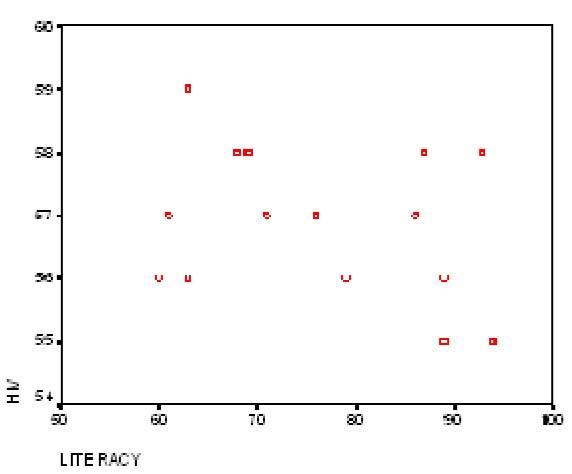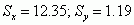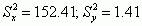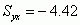# Study Questions

The following set of questions utilizes data from the Population Reference Bureau (PRB) on the relationship between women’s literacy and the prevalence of HIV in Sub-Saharan Africa. Using the PRB’s Data Finder tool, data were assembled for 15 countries in Sub-Saharan Africa through the year 2004 and are shown below.

 Country Female Literacy Rate Percent Women with HIV Angola 59 63 Botswana 58 93 Democratic Congo 57 61 Djibouti 56 79 Eritrea 56 60 Madagascar 58 68 Malawi 57 71 Namibia 55 94 Nigeria 58 87 Rwanda 57 76 Sudan 58 69 Swaziland 55 89 Tanzania 56 89 Togo 56 63 Zambia 57 86

1. A researcher advances the hypothesis that women in countries with lower female literacy rates tend to see a greater percentage of the female population infected with HIV. What is the independent variable here? What is the dependent variable here?

2. Using the above data, a scatter diagram was constructed. Very generally, what does this scatter diagram indicate about the relationship between female literacy rates and the percentage of females with HIV? Does the relationship appear to be negative, positive, or neither? Interpret this relationship (assuming it was the true relationship in the population).3. Several statistics were calculated from these data for both the independent and the dependent variables. Identify (i.e., name) the statistic summarizing the independent and dependent variables below.

1.2.3.4.4. Without doing any calculations, you should be able to identify the direction of the relationship between female literacy rates and the percentage of females with HIV from the statistics provided in Question #3. What is the direction of this relationship?

5. Using the statistics provided in Question #3, calculate both the Y-intercept and slope coefficient for the regression equation. Once you arrive at these quantities, write down the full regression equation in proper notation and provide a one-sentence interpretation of the slope coefficient.

6. According to the regression equation that you calculated above, what is the predicted percentage of women with HIV in a hypothetical country where the female literacy rate is equal to zero?

7. Using the statistics provided in Question #3, calculate the value of Pearson’s correlation coefficient, r, and provide a one-sentence interpretation of this quantity. Likewise, calculate the value of the coefficient of determination, r2, and provide a one-sentence interpretation of this quantity.

8. According to the above regression equation, what percentage of variation in the dependent variable—the percentage of females with HIV—is explained by only considering the independent variable—female literacy rates? What is the statistical term for this quantity as it was referred to in Chapter 12 of your textbook. What percentage of variation remains unexplained?

9. The regression sum of squares reflects the improvement in the prediction error resulting from the use of the linear regression prediction equation. Calculate the value of the regression sum of squares if r2 = .09 and the sum of squares total = 19.73.

10. For each of the 15 countries considered in this analysis, what is the predicted value for the percentage of women with HIV? Identify the five countries with the largest residuals? Pick one of these countries and interpret its residual in statistical terms.

11. Suppose that a researcher added as an additional independent variable the percentage of women who work outside of the home to the original bivariate regression equation. Describe what you think would happen to the following value: Σe2.

12. The equation, Ÿ= a + bx, is the general form for a bivariate regression equation. What would be the general form of the equation to summarize the addition of the percentage of women who work outside of the home to the original bivariate regression equation? Write this equation down and provide a statistical interpretation of each quantity in this regression equation?